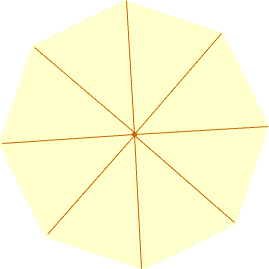Quandaries and Queries If i have a measure of one interior angle of a polygon, how can i find the number of sides it has?  Ernie Secondary Hi Ernie, I assume you are refering to a regular polygon, one where all the sides are the same length and all the external angles are congruent. Otherwise the number of sides does not determine the measures of the angles. In a regular polygon with n sides, join each vertex to the center to form n triangles.For each triangle the angle at the center has measure 360/n degrees. Since the sum of the angle measures in any triangle is 180 degrees, in each of the triangles the sum of the other two angle measures is 180 - 360/n degrees.Thus each interior angle in the polygon has measure 180 - 360/n degrees. Penny Go to Math Central